Transforming Numerical Methods Education for the STEM Undergraduate MOOC | MOBILE | VIDEOS | BLOG | YOUTUBE | TWITTER | COMMENTS | ANALYTICS | ABOUT | CONTACT | COURSE WEBSITES | BOOKS | MATH FOR COLLEGE
 MULTIPLE CHOICE TEST RUNGE-KUTTA 4TH ORDER METHOD ORDINARY DIFFERENTIAL EQUATIONS

Q1. To solve the ordinary differential equation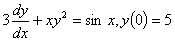,

by Runge-Kutta 4th order method, you need to rewrite the equation as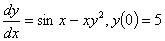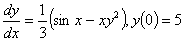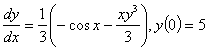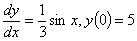Q2. Given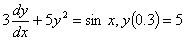and using a step size of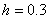, the value of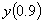using Runge-Kutta 4th order method is most nearly

- 0.25011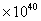-4297.4

-1261.5
0.88498
Note: At the end of this document, see formulas used to answer this question as there are a few different versions of the Runge-Kutta 4th order method.

Q3. Given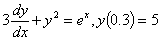, and using a step size of, the best estimate ofRunge-Kutta 4th order method is most nearly

-1.6604
-1.1785
-0.45831
2.7270

Note: At the end of this document, see formulas used to answer this question as there are a few different versions of the Runge-Kutta 4th order method.

Q4. The velocity (m/s) of a parachutist is given as a function of time (seconds) by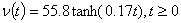Using Runge-Kutta 4th order method with a step size of 5 seconds, the distance traveled by the body from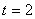to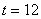seconds is estimated most nearly as

341.43 m

428.97 m

429.05 m

703.50 m

Note: At the end of this document, see formulas used to answer this question as there are a few different versions of the Runge-Kutta 4th order method.

Q5.  Runge-Kutta method can be derived from using first three terms of Taylor series of writing the value of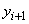, that is the value of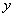at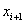,  in terms of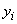and all the derivatives ofat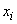.  If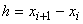, the explicit expression forif the first five terms of the Taylor series are chosen for the ordinary differential equation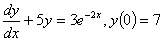,

would be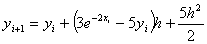Q6. A hot solid cylinder is immersed in an cool oil bath as part of a quenching process. This process makes the temperature of the cylinder,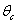, and the bath,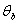, change with time.  If the initial temperature of the bar and the oil bath is given as 600 C and 27C, respectively, andLength of cylinder = 30 cm

Radius of cylinder = 3 cm

Density of cylinder = 2700 kg/m^3

Specific heat of cylinder = 895 J/kg-K

Convection heat transfer coefficient = 100 W/(m^2-K)

Specific heat of oil = 1910 J/(kg-K)

Mass of oil = 2 kg

The coupled ordinary differential equations governing the heat transfer are given by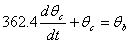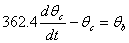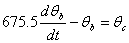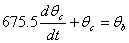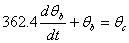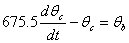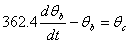The following equations are used to answer questions#2, 3, and 4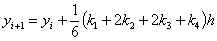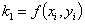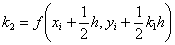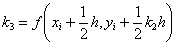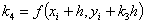AUDIENCE |  AWARDS  |  PEOPLE  |  TRACKS  |  DISSEMINATION  |  PUBLICATIONS Copyrights: University of South Florida, 4202 E Fowler Ave, Tampa, FL 33620-5350. All Rights Reserved. Questions, suggestions or comments, contact kaw@eng.usf.edu  This material is based upon work supported by the National Science Foundation under Grant#0126793, 0341468, 0717624,  0836981, , 0836805, 1322586.  Any opinions, findings, and conclusions or recommendations expressed in this material are those of the author(s) and do not necessarily reflect the views of the National Science Foundation.  Other sponsors include Maple, MathCAD, USF, FAMU and MSOE.  Based on a work at http://mathforcollege.com/nm.  Holistic Numerical Methods licensed under a Creative Commons Attribution-NonCommercial-NoDerivs 3.0 Unported License. ANALYTICS